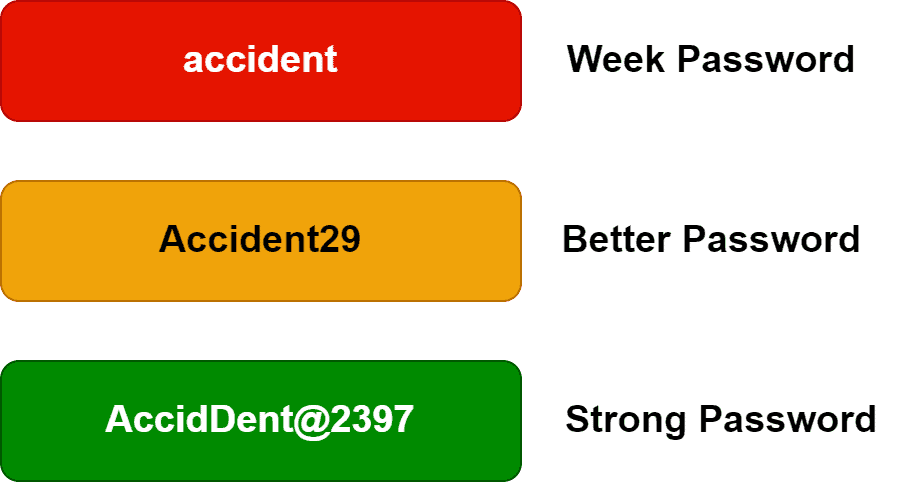## 1. Overview

In this tutorial, we’ll discuss the calculation of password entropy with an example.

## 2. Introduction to Password Entropy

Password plays a vital role in preserving the privacy and security of digital systems and users. Furthermore, it prevents unauthorized access to personal digital information and digital devices. Additionally, it helps in authentication and safeguards the integrity as well as the confidentiality of data.

Now when setting a password, it’s crucial to choose a strong or safe password. Therefore, one way to check whether a password is safe or not is by measuring its strength. Hence, a good way to determine the strength of a password is to estimate its length. Furthermore, we can do this by counting the number of characters in a password.

Moreover, a password is secure if it’s 12-14 characters long. Additionally, in order to increase the strength of the password, it’s advised to use a combination of lowercase letters, uppercase letters, numbers, and symbols. Furthermore, let’s look at some examples of various types of passwords:Importantly, given a password, it’s essential to determine how secure it is for use in the digital world. Entropy is the method to measure the quality of a password. Furthermore, a higher entropy value denotes a stronger password.

## 3. Password Strength with Cracking Odds

Generally, a password is secure or robust if it’s hard to crack. Hence, the time required for cracking a password indicates how secure a password is. Furthermore, let’s explore how long it takes to crack various passwords.

Cracking odds are the chances of a password being cracked concerning the number of possible combinations. For example, a password with 3 characters containing only uppercase letters has less than 20000 possible combinations. Therefore, a simple brute-force attack can easily break the password.

Let’s take some examples and explore the cracking time of passwords with respect to cracking odds. Based on the research analysis, it’s stated that a 7-character long password that contains only uppercase or lowercase letters can be cracked instantly. However, a 10-character long password that contains at least one uppercase letter, one lowercase letter, one number, and one symbol takes 5 years to crack.

Hence, the password’s strength increases exponentially with a higher number of characters. Additionally, mixing a password with uppercase letters, lowercase letters, numbers, and symbols boost its strength.

## 4. Calculation of Password Entropy

Using a complex password is a good way to protect a digital account from hackers. However, it might not be enough. Therefore, we need to ensure that the selected password has high entropy. A high entropy indicates a high amount of variation in the selected password. Hence, a longer password with a large variation has higher entropy, making it harder to crack.

Based on the entropy score, we can divide a password into four categories: poor, weak, reasonable, and very good:When determining the entropy of a password, we first need to find out the number of possible combinations of characters the password could have.

Now let’s take a look at the formula for finding the entropy of a password:Here E denotes password entropy value. Furthermore, the variable R is the range of characters. The password length is indicated by L. Finally, the logarithm operation determines the total number of possible combinations.

Now let’s take an example password and calculate the entropy score. Specifically, we’re taking a 10-digit long password that contains uppercase, lowercase, and numbers: Better2023. Here, the password length L = 10. As the password contains uppercase, lowercase, and numbers, the rage of the characters R = 26 + 26 + 10 = 62. Therefore, it means thatguesses are required to ensure that the password is discovered.

Let’s calculate the entropy score:## 5. Conclusion

In this tutorial, we discussed the calculation of password entropy with an example.